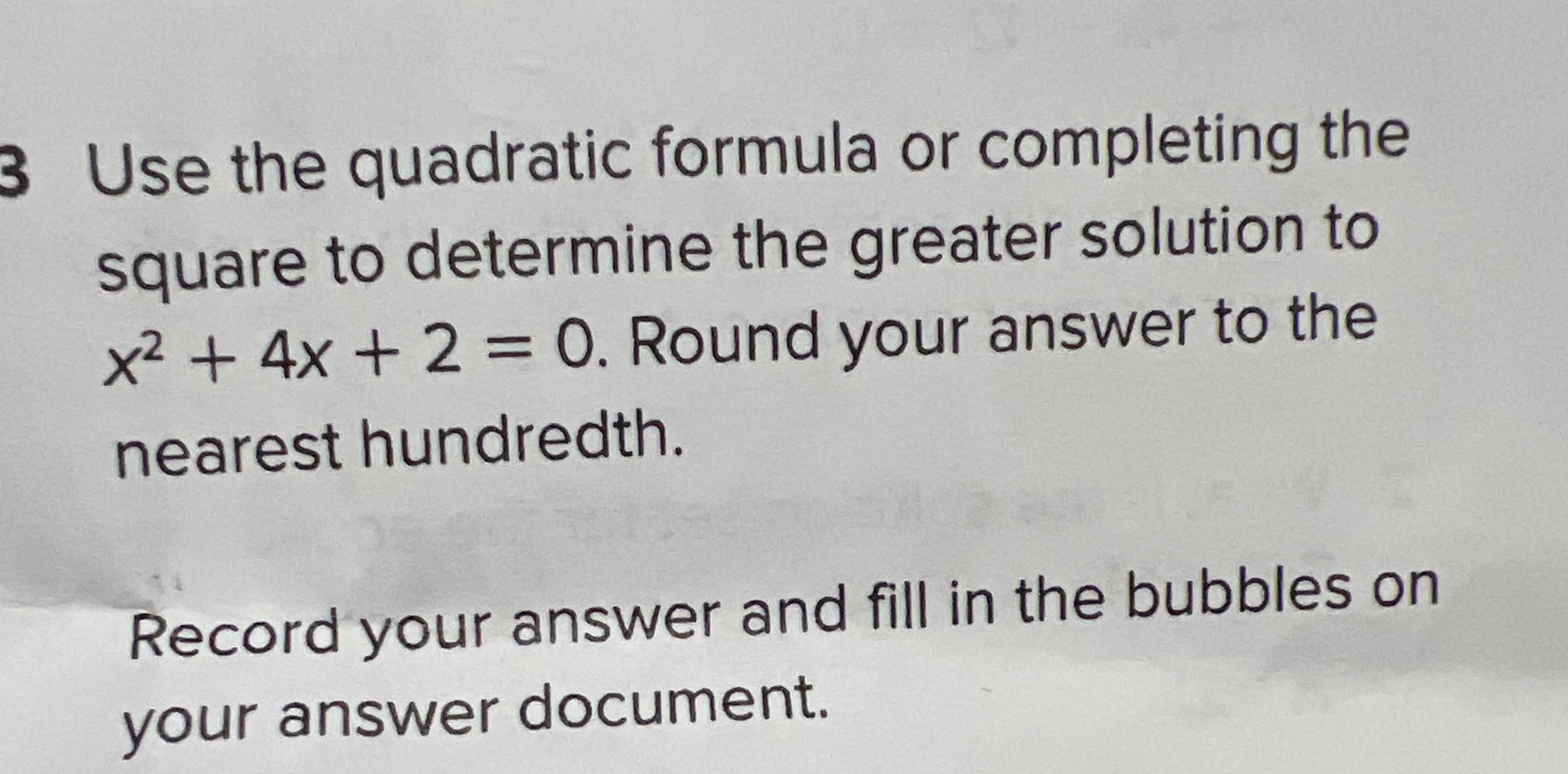### ¿Todavía tienes preguntas de matemáticas?

Pregunte a nuestros tutores expertos
Algebra
Pregunta$$3$$ Use the quadratic formula or completing the square to determine the greater solution to

$$x ^ { 2 } + 4 x + 2 = 0$$ . Round your answer to the nearest hundredth. Record your answer and fill in the bubbles on your answer document.

x=$$\frac{- 4\pm2\sqrt{2} }{2} = -2\pm \sqrt{2}$$